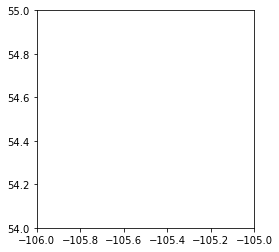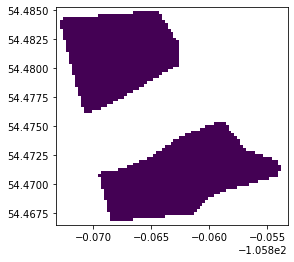# disturbances_2012_moja#

You can find instructions to open a geoTIFF file here. Instructions on how to download and install Rasterio and GDAL(not necessary for linux) are included too.

In addition to that, we also need to install matplotlib and Shapely in order to clip the raster.

To install matplotlib and Shapely, run the commands :

```pip install matplotlib
```
```pip install shapely
```

Import the required libraries

```import rasterio as rst
from rasterio.plot import show
import matplotlib.pyplot as plt
import numpy as np
```

Store the geoTIFF file in a variable, use the rs.open() and show() functions to open view the image in the next cell.

We can also perform different operations using raster objects: the number of bands, the image resolution, CRS value, no-data value, number of raster bands, etc.

```disturbances12 = r'disturbances_2012_moja.tiff'
img = rst.open(disturbances12)
show(img)
#No. of bands
print(img.count)

#Image resolution
print(img.height, img.width)

# CRS
print(img.crs)

# No-data
print(img.nodata)
``````1
4000 4000
EPSG:4326
255.0
```

The image has a lot of padding around the area of interest, hence we need to clip the raster.

```from rasterio.features import dataset_features
```

dataset_features yields GeoJSON-like feature dictionaries for shapes found in the given band

Using this, we get the bounding box from all shapes in the output

```geom_gen = dataset_features(img, bidx=1)
bboxes = [geom['bbox'] for geom in geom_gen]
bboxes
```
```[[-105.873, 54.47575, -105.8625, 54.485],
[-105.86975, 54.46675, -105.8535, 54.4755]]
```

Next, we assign the extremums from the bounding box to different variables

```left   = min([bbox for bbox in bboxes])
bottom = min([bbox for bbox in bboxes])
right  = max([bbox for bbox in bboxes])
top    = max([bbox for bbox in bboxes])
```

Create a Polygon geometry for the mask

```from shapely.geometry import box

# create a POLYGON geometry for the mask
geom = box(left, bottom, right, top)
geom.wkt
```
```'POLYGON ((-105.8535 54.46675, -105.8535 54.485, -105.873 54.485, -105.873 54.46675, -105.8535 54.46675))'
```

```from rasterio.mask import mask
```

Assigning the data for the clipped image

```out_image, out_transform = mask(img, [geom], crop=True, pad=True)
out_meta = img.meta
```
```out_meta.update({"driver": "GTiff",
"height": out_image.shape,
"width": out_image.shape,
"transform": out_transform})

with rst.open(r'disturbances_2012_moja_masked.tiff', 'w', **out_meta) as dest:
dest.write(out_image)
```

View the clipped raster

```fp_result = r'disturbances_2012_moja_masked.tiff'
result = rst.open(fp_result)
show(result)
``````<AxesSubplot:>
```

View JSON data parsed from disturbances_2012_moja

```import json
```
```with open("disturbances_2012_moja.json") as f:
data
```
```{'layer_type': 'GridLayer',
'layer_data': 'Byte',
'nodata': 255,
'tileLatSize': 1.0,
'tileLonSize': 1.0,
'blockLatSize': 0.1,
'blockLonSize': 0.1,
'cellLatSize': 0.00025,
'cellLonSize': 0.00025,
'attributes': {'1': {'year': 2012,
'disturbance_type': 'Wildfire',
'transition': 1}}}
```